# How to convert RGBA Amber to HSV

Some time ago, I obtained some LED par lamps that had amber LEDs in addition to the usual red, green and blue. This makes for generally warmer oranges and yellows as it increases the color gamut available. To control the colors in a natural way, I wrote a little routine to squeeze the orange hues into the usual color wheel (hue, saturation, value) representation. More details can be found in this blog post: /2015/Generating-RGBY-from-Hue/.

Fast-forward several years when someone asked me if the reverse transformation is possible. Turns out it is, but it's definitely more complicated and not at all perfect. (This is to be expected, as we are converting from a four-dimensional (R, G, B, A) space to the three dimensions of H, S, V so naturally the solution is only an approximation.)

To do this, I used the perceptually-based CIE xyY to model what color a mix of RGBA primaries will yield. Each primary light source is represented as a point in `xy` space, and they can be interpolated to find the `xy` coordinates of the resulting mixture. Once this is determined, the `xy` coordinates can be converted to HSV.

Here's an example of how that interpolation works: I've generated a ring of colors of different hues and similar saturation by appropriately mixing R, G, and B primaries. Mixing in a successively brighter amber LED shifts the colors towards the amber primary at 590nm.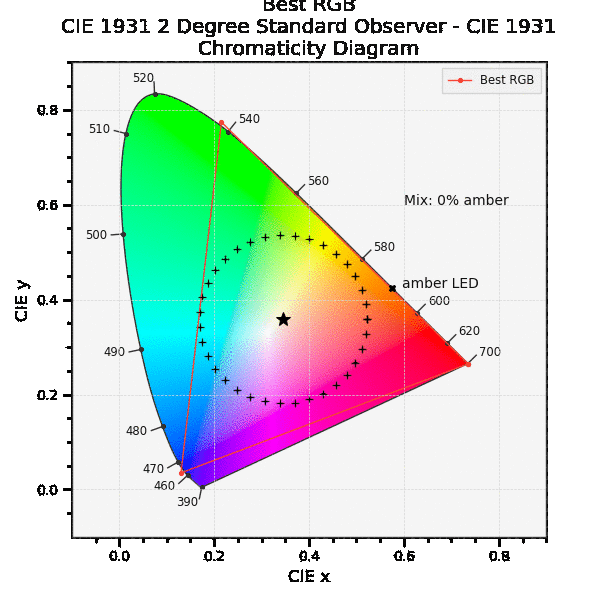Here's a library to do the color space conversions on Github: github.com/headrotor/colorxform/ Details on the various conversions follow.

The `HSV_to_RGBA()` and `HSV_to_RGBA_CIE()` methods divide the hue range into four sections corresponding to red, amber, green, and blue, and crossfades between them to arrive at an approximately correct hue.

The first `HSV_to_RGBA()` is the straightforward approach where the amber values are squeezed in between the red and green (further described in this blog post: /2015/Generating-RGBY-from-Hue/).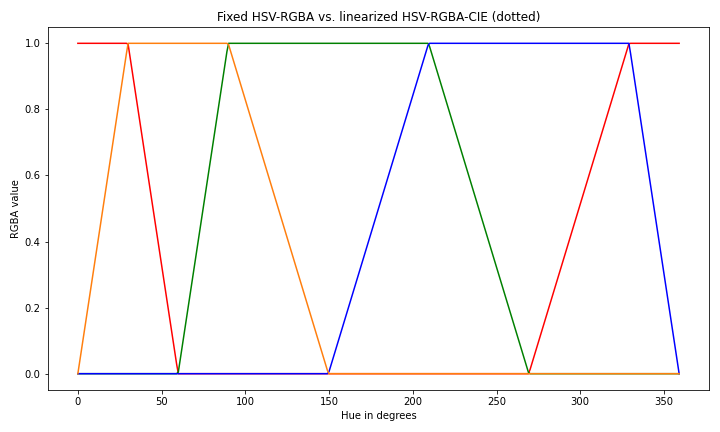A more sophisticated approach `HSV_to_RGBA_CIE()` gives colors that are closer to equal angles in `xy` space and should thus be a perceptually smoother transition around the hue circle.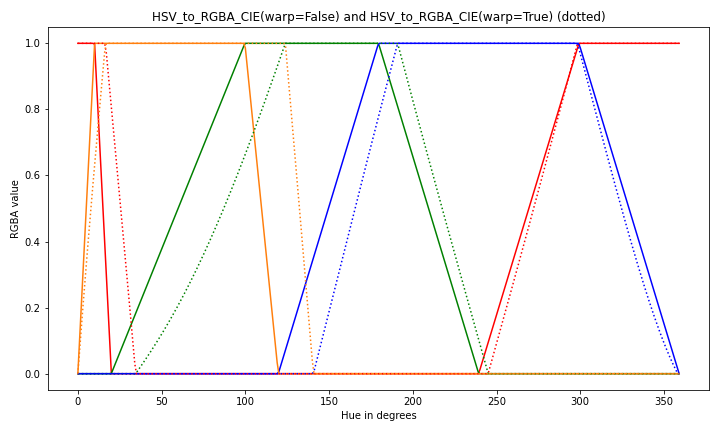A benefit of the CIE approach is that a round-trip conversion `HSV``RGBA``HSV` will result in hues that are much closer to the original. The `HSV_to_RGBA_CIE()` method also has an optional parameter `warp` that when set to true (the default) will prewarp the hue to better match the `RGBA_to_HSV_CIE()` results. `warp` uses a 6th-order polynomial fit to warp the input hue value; if round-trip hue matching is of less importance then `warp` mat be set to False to skip this computation.

The following image shows the locus in `xy` space of hues equally spaced in 10 degree increments given by `HSV_to_RGBA()` on the left and `HSV_to_RGBA_CIE()` on the right. Note that the CIE version is more regularly spaced and so should be more perceptually equal as well.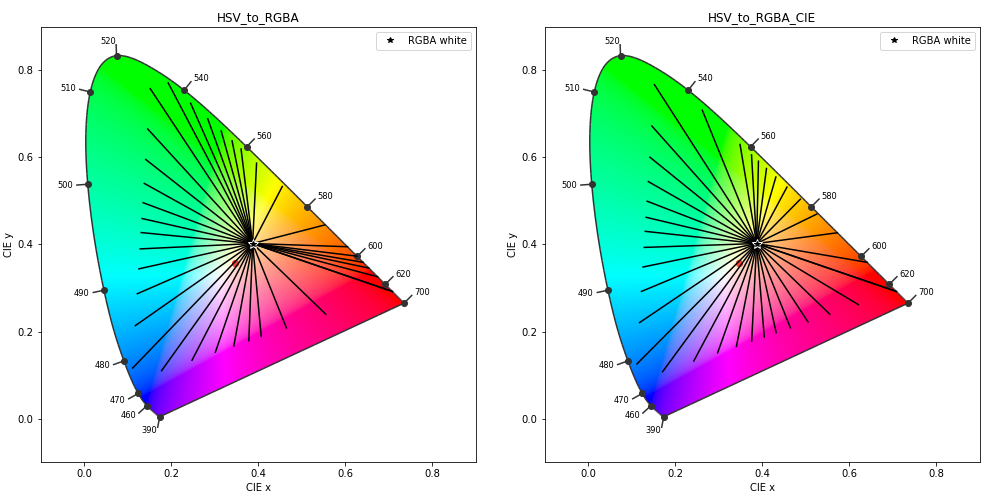The `RGBA_to_HSV_CIE()` function is somewhat more complex and is naturally an approximation as we are converting from a four-dimensional space to three. Each light source (R, G, B, and A) is converted into into the CIE xy color coordinates, and interpolated to find the color of the resulting mixture. Once this is calculated, the `xy` coordinates can be converted to HSV.

To convert from `xy` coordinates to HSV, we need to determine the hue, saturation, and value. The saturation can be obtained by the scaled distance from the CIE white point (the ★ in the animation above) to the `xy` value, but in practice it is simpler to just use the minimum of the RGBA values. The value, or brightness, is not specified in `xy` space so the maximum of the RGBA values is used. Here is how this works for HSV-RGB, the extension to RGBA is straightforward.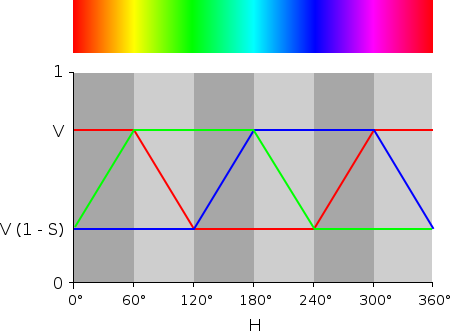To calculate the hue, we need to find the angular distance around the hue circle. in `xy` space, this is pretty well captured by the angle around the white point. For example, given an arbitrary green color (labeled `g` here), the hue angle is the angle between vector `g` (solid) and the red primary vector (dotted). So the hue angle for an arbitrary color is calculated from the angle in `xy` space by reversing the direction (so increasing hue goes sequentially from red to green to blue) and subtracting the red angle (so zero hue corresponds to red).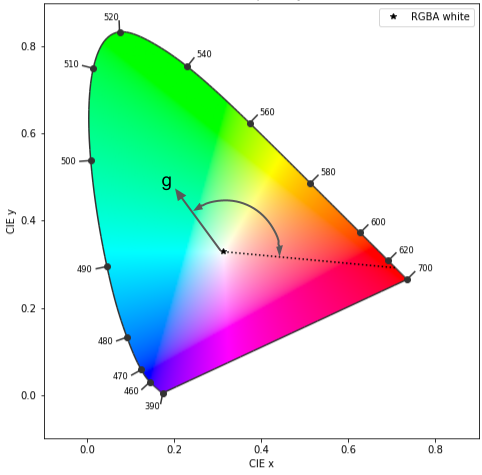Using the CIE conversion functions and warping reduce the round-trip hue error to less than a few percent (e. g. `HSV_to_RGBA_CIE(warp=True)``RGBA_to_HSV_CIE()`), as shown by the green line in the following figure.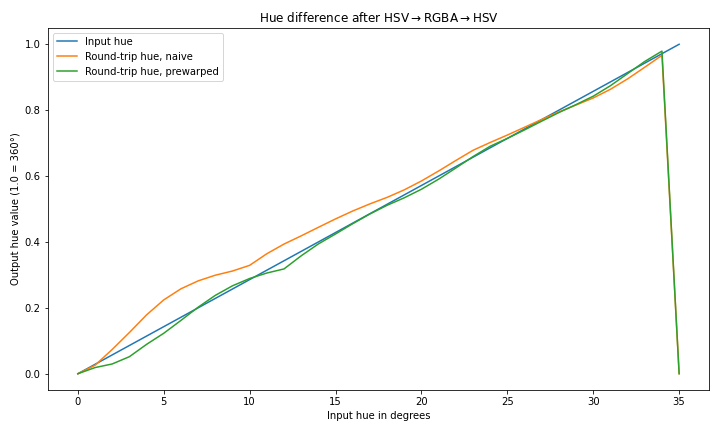The warping is accomplished using a 6-degree polynomial fit to the difference between the input hue and output hue.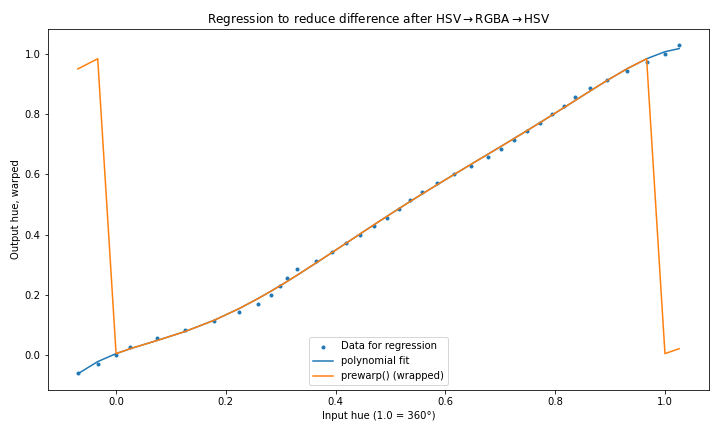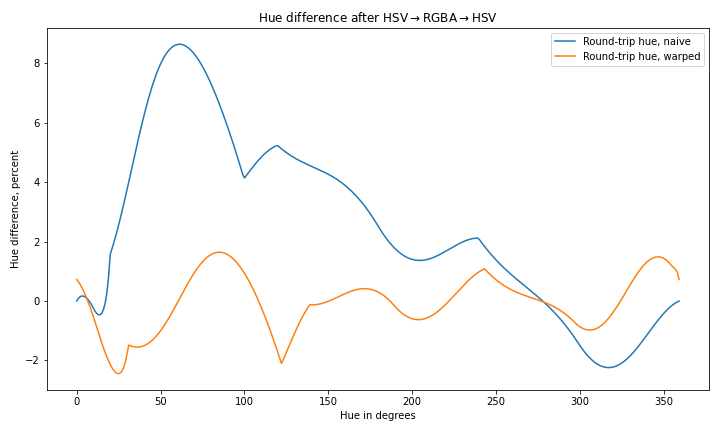Note 1: color-space nomenclature is confusing! Colorspace names are in uppercase except `xyY` to disambiguate `X`, `Y` from `x`, `y` from CIE `xyY` space. Four-color space is `RGBA` with `A` for Amber. (Though `Y` is often used for for amber/Yellow to disambiguate from alpha channel "A", we use `A` here to disambiguate from `Y` in `xyY` and `XYZ` color spaces.)

Tagged: color math

# Topics: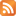RSS feed